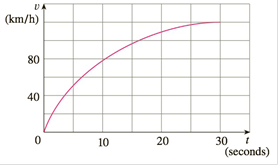Chapter 4.1, Problem 18E

Chapter
Section
Textbook Problem

# The velocity graph of a car accelerating from rest to a speed of 120 km/h over a period of 30 seconds is shown. Estimate the distance traveled during this period.To determine

To estimate:

The distance traveled

Explanation

1) Concept:

Area under the graph is

A=limni=1nfxix

where,

x=b-an & xi=a+ix

2) Calculation:

The velocity graph of the car accelerating from rest to a speed of

120kmh

over a period of 30 seconds.

So, the interval 0, 30 with n=6.

Find out  x.

x=b-an=30-06=5

For an increasing function, using left end points gives an underestimate and using right end points gives an overestimate

### Still sussing out bartleby?

Check out a sample textbook solution.

See a sample solution

#### The Solution to Your Study Problems

Bartleby provides explanations to thousands of textbook problems written by our experts, many with advanced degrees!

Get Started

#### Find more solutions based on key concepts